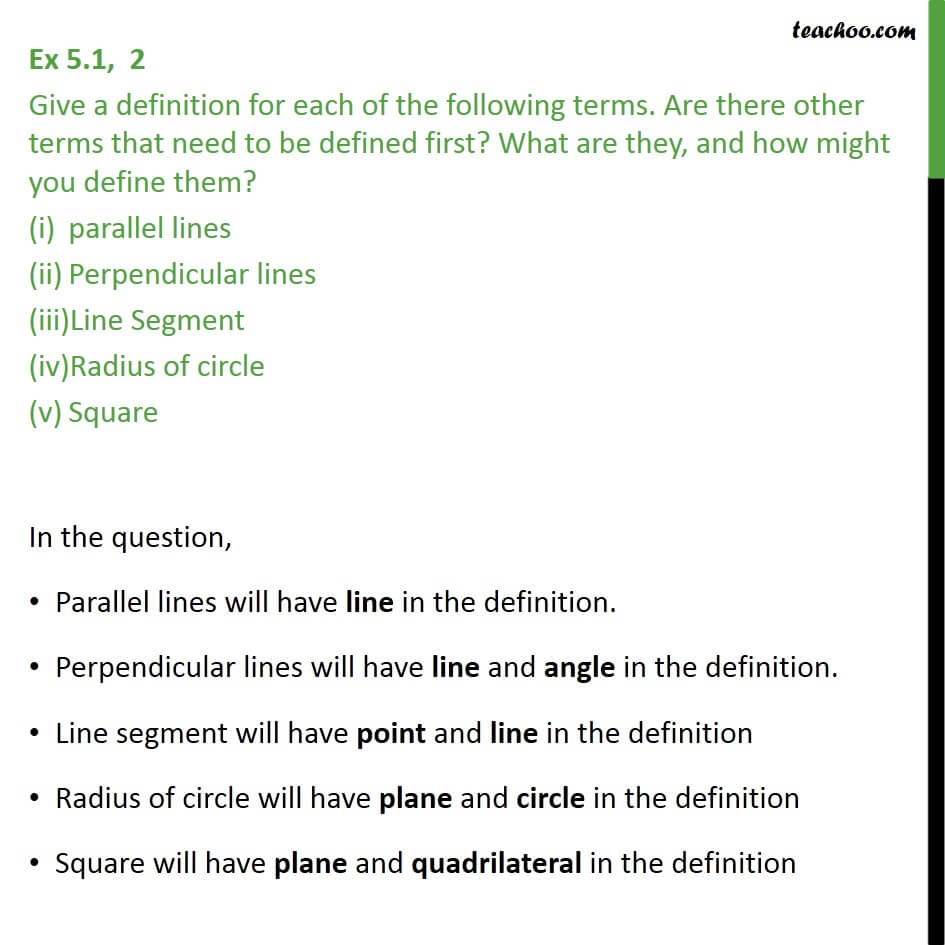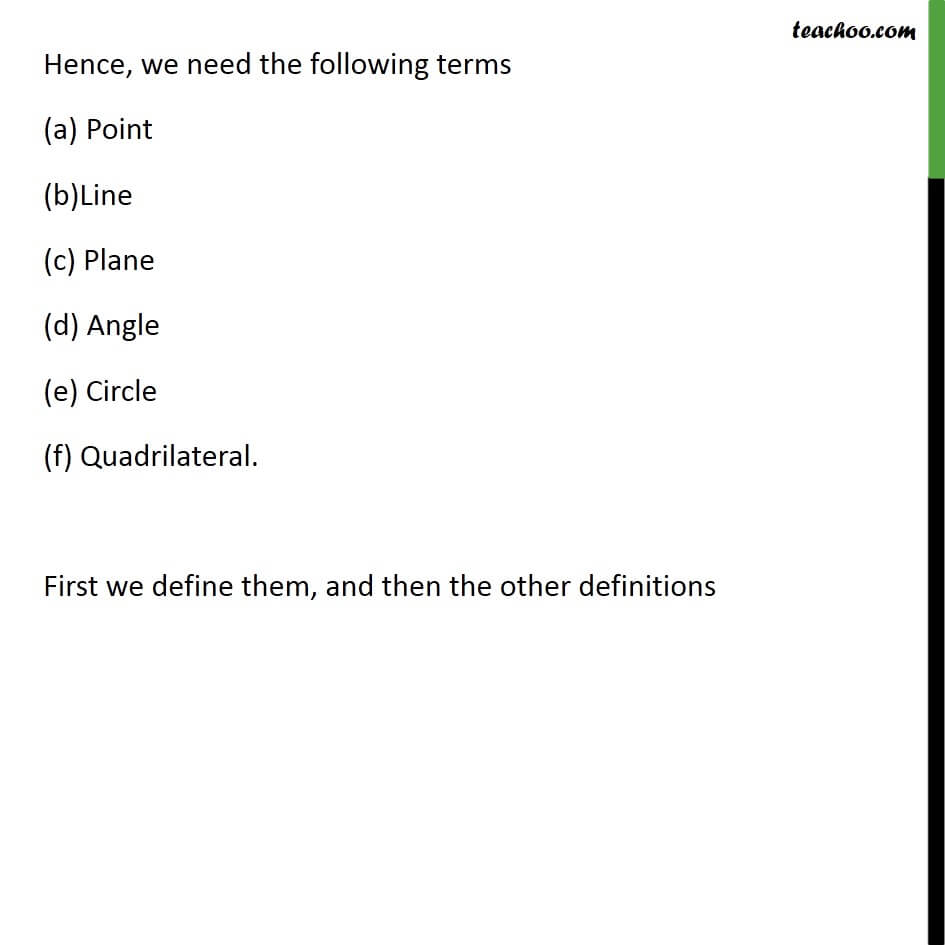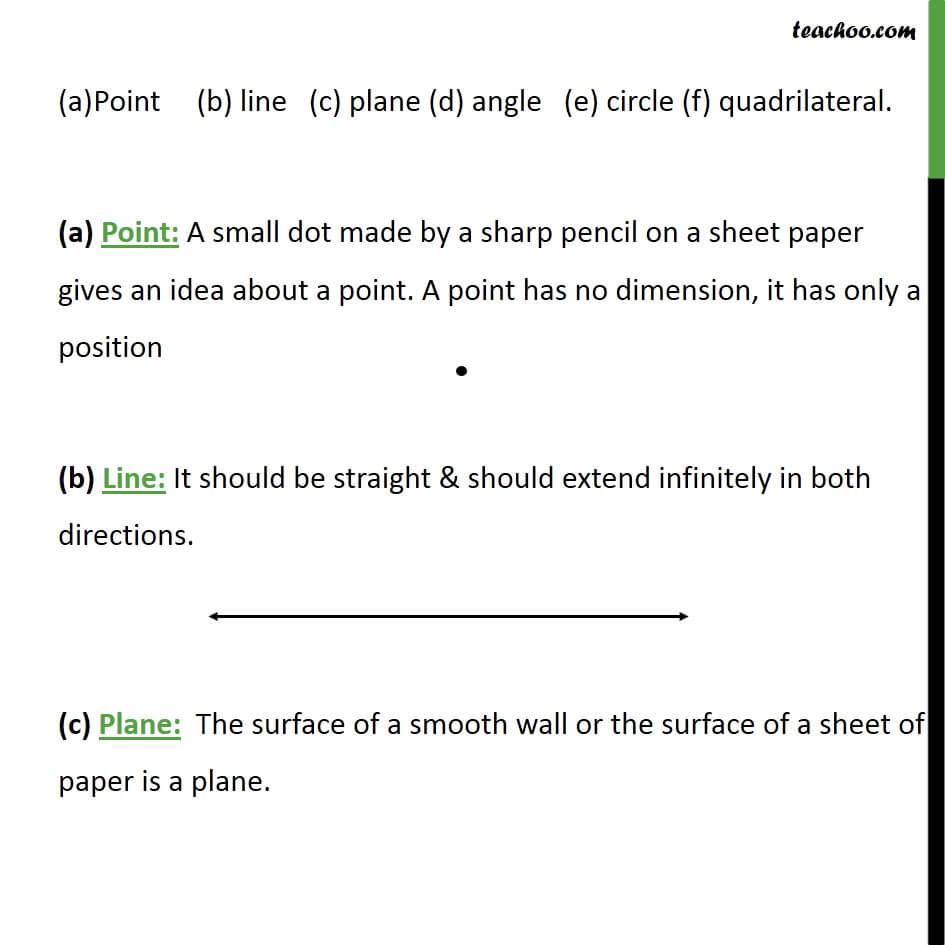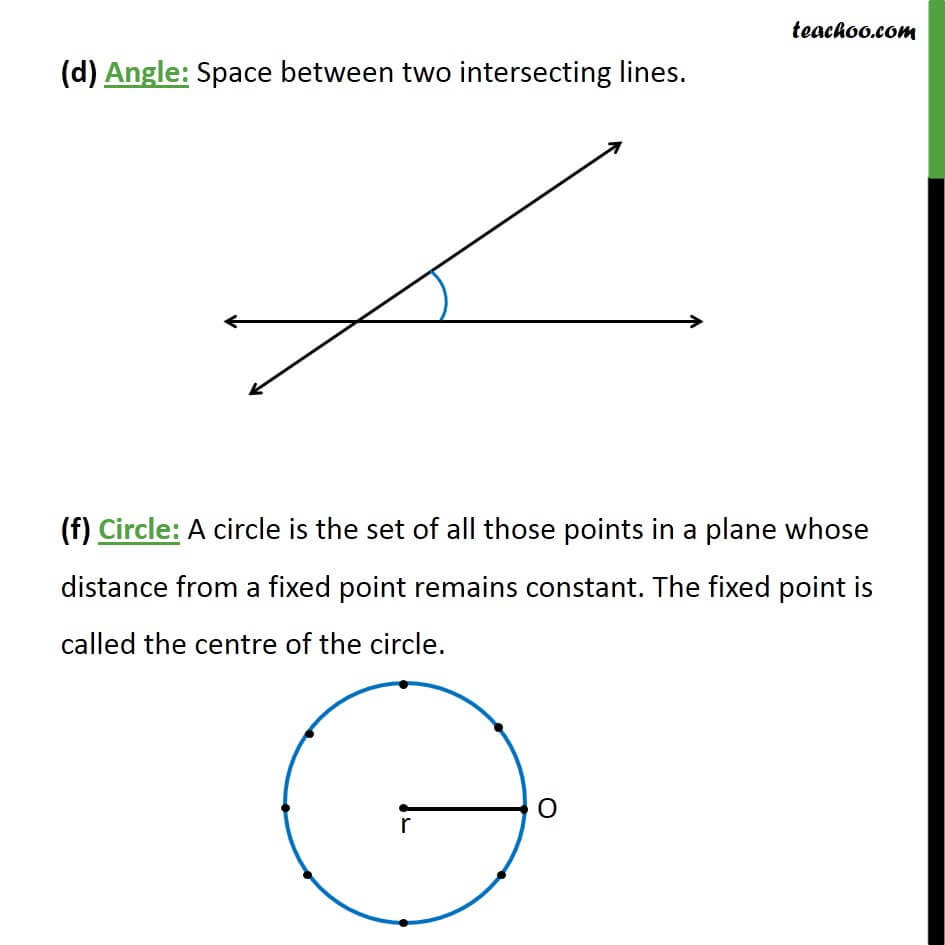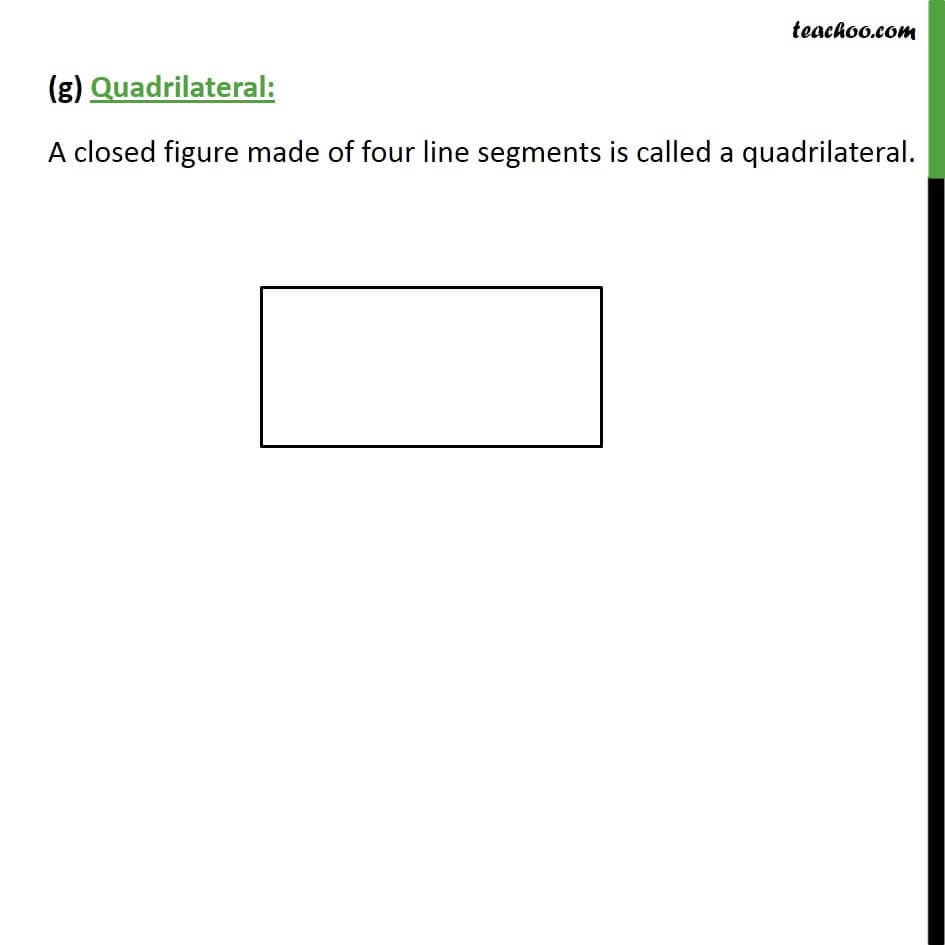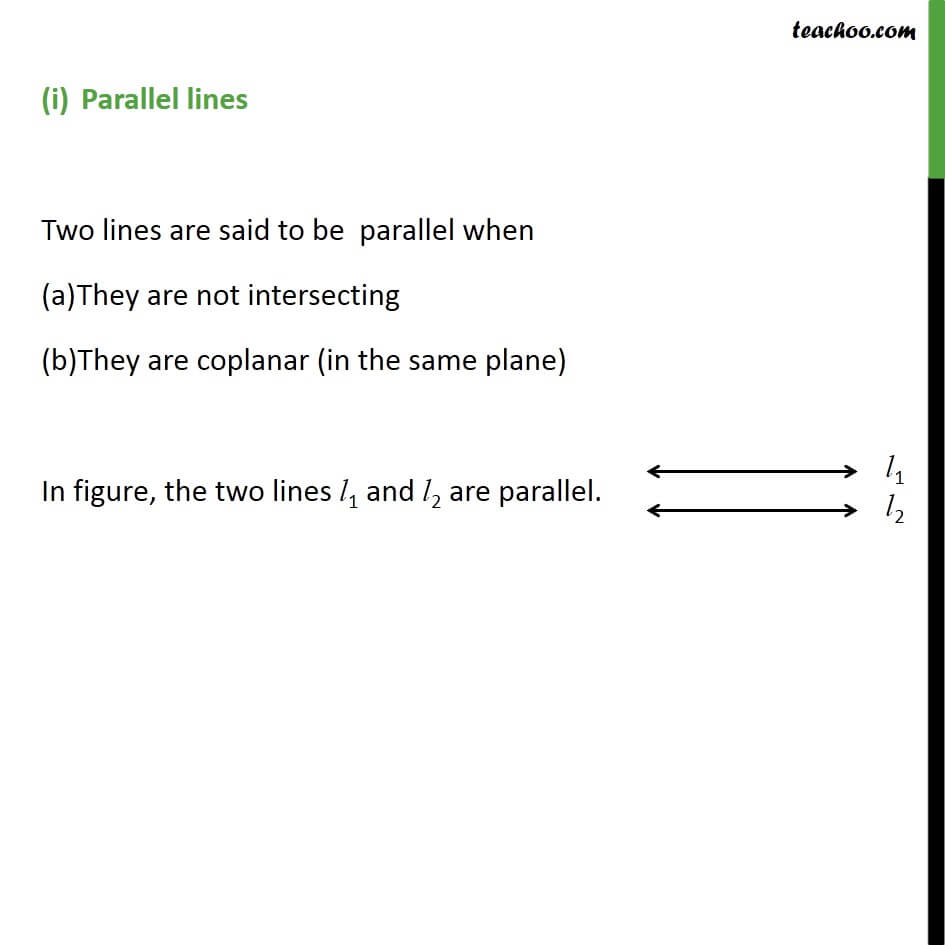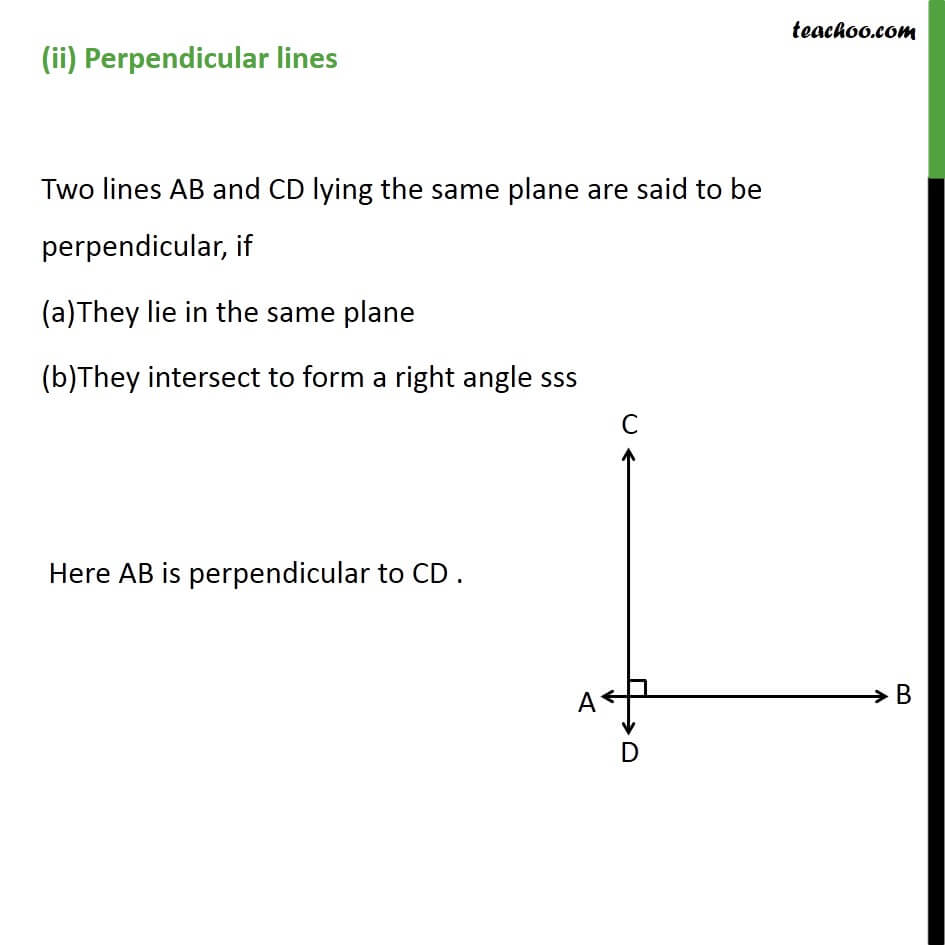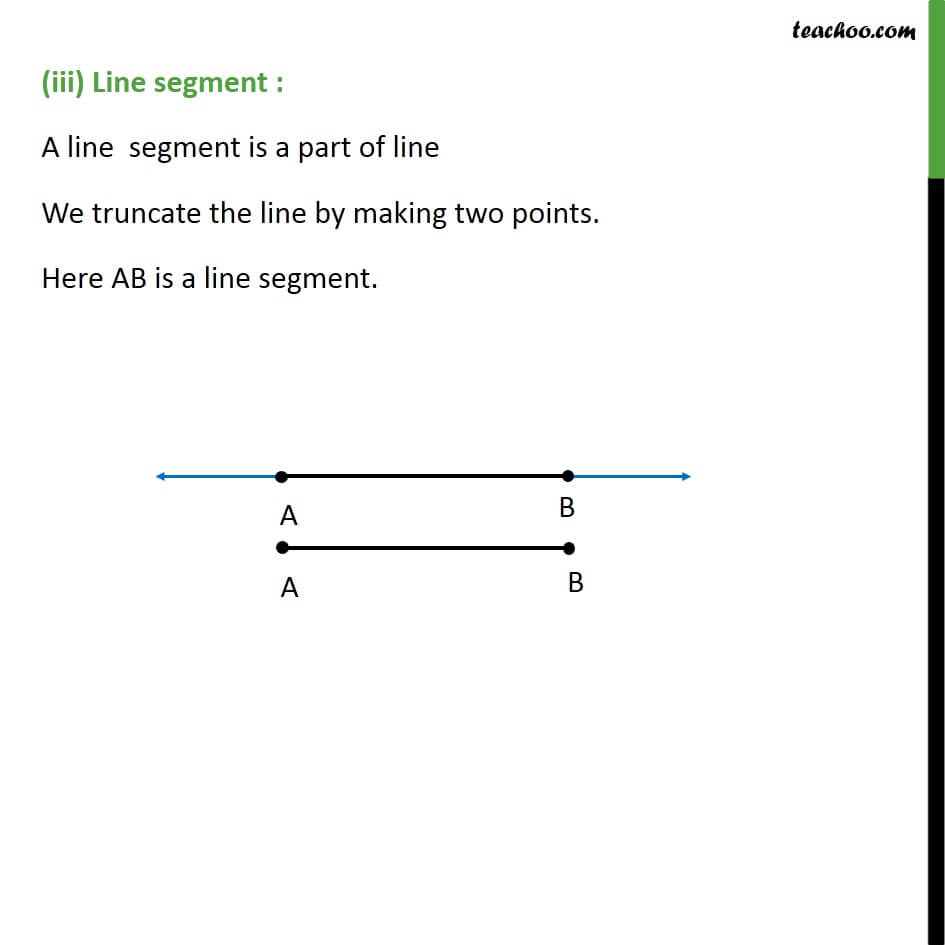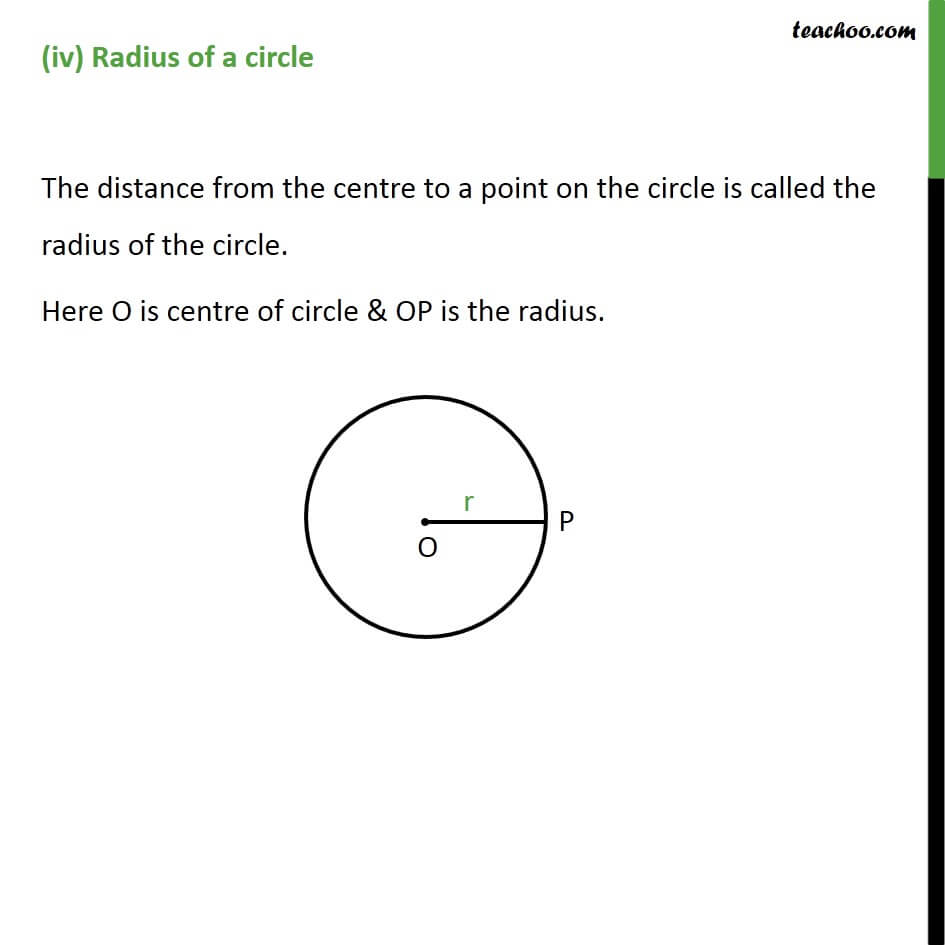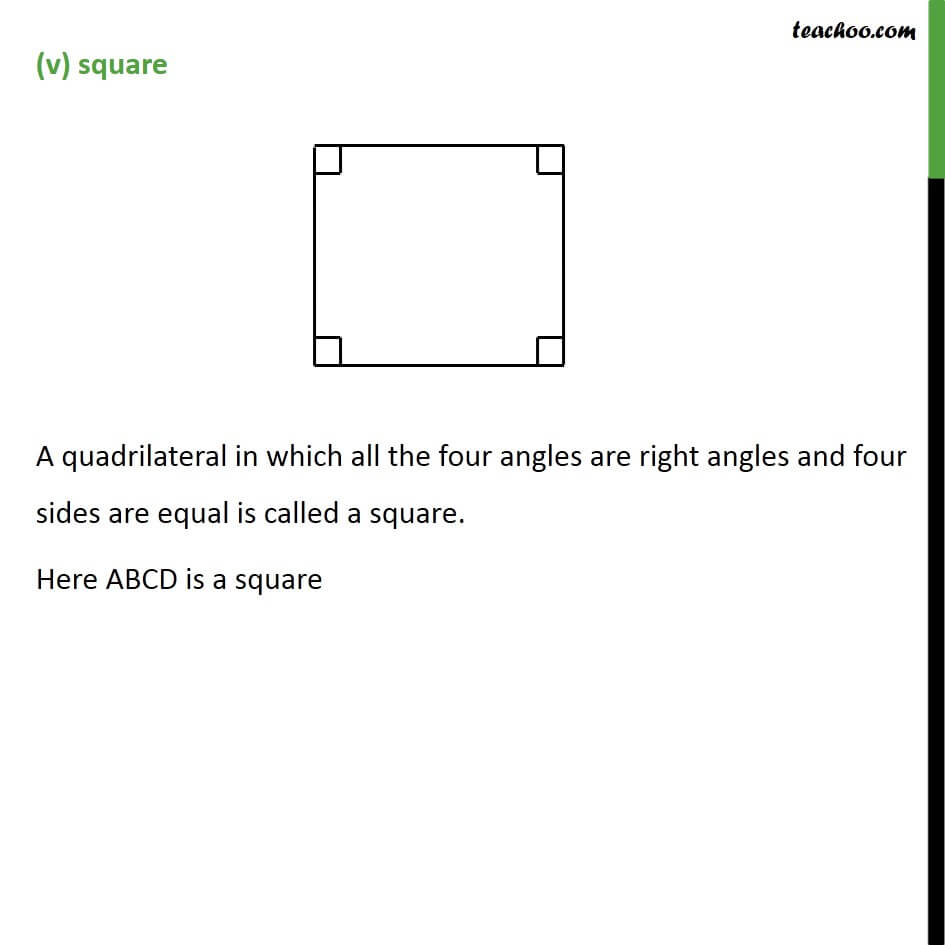1. Chapter 5 Class 9 Introduction to Euclid's Geometry (Deleted)
2. Serial order wise
3. Ex 5.1

Transcript

Ex 5.1, 2 Give a definition for each of the following terms. Are there other terms that need to be defined first? What are they, and how might you define them? parallel lines Perpendicular lines Line Segment Radius of circle Square For the desired definition, we need the following terms : Point Line (c) Plane (d) Angle (e) Circle (f) Quadrilateral. First we define them, and then the other definitions Point (b) line (c) plane (d) angle (e) circle (f) quadrilateral. (a) Point: A small dot made by a sharp pencil on a sheet paper gives an idea about a point. A point has no dimension, it has only a position (b) Line: It should be straight & should extend infinitely in both directions. (c) Plane: The surface of a smooth wall or the surface of a sheet of paper is a plane. (d) Angle: Space between two intersecting lines. (f) Circle: A circle is the set of all those points in a plane whose distance from a fixed point remains constant. The fixed point is called the centre of the circle. (g) Quadrilateral: A closed figure made of four line segments is called a quadrilateral. Ex 5.1, 2 Give a definition for each of the following terms. Are there other terms that need to be defined first? What are they, and how might you define them? Parallel lines Two lines are said to be parallel when They are not intersecting They are coplanar (in the same plane) In figure, the two lines L1 and L2 are parallel. Ex 5.1, 2 Give a definition for each of the following terms. Are there other terms that need to be defined first? What are they, and how might you define them? (ii) Perpendicular lines Two lines AB and CD lying the same plane are said to be perpendicular, if They lie in the same plane They intersect to form a right angle sssHere AB is perpendicular to CD . Ex 5.1, 2 Give a definition for each of the following terms. Are there other terms that need to be defined first? What are they, and how might you define them? (iii) Line segment Line segment : A line segment is a part of line We truncate the line by making two points. Here AB is a line segment. Ex 5.1, 2 Give a definition for each of the following terms. Are there other terms that need to be defined first? What are they, and how might you define them? (iv) Radius of a circle The distance from the centre to a point on the circle is called the radius of the circle. Here O is centre of circle & OP is the radius. Ex 5.1, 2 Give a definition for each of the following terms. Are there other terms that need to be defined first? What are they, and how might you define them? (v)square A quadrilateral in which all the four angles are right angles and four sides are equal is called a square. Here ABCD is a square

Ex 5.1